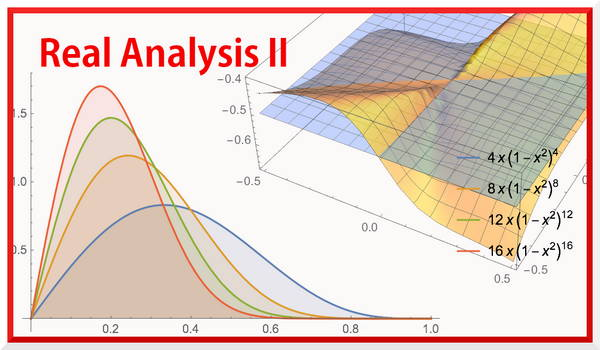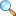# MTH322: Real Analysis II (Spring 2023)This course is offered to BS, Semester VI at Department of Mathematics, COMSATS University Islamabad, Attock campus. This course need rigorous knowledge of continuity, differentiation, integration, sequences and series of numbers, that is many notions included in Real Analysis I.

Sequences of functions: Convergence, uniform convergence, uniform convergence and continuity, uniform convergence and integration, uniform convergence and differentiation, the exponential and logarithmic function, the trigonometric functions.

Series of functions: Absolute convergence, uniform convergence, Cauchy criterion, Weiestrass M-test, power series of functions, radius of convergence, Cauchy-Hadamard theorem, differentiation theorem, uniqueness theorem.

Improper integrals: Improper integral of first and second kind, comparison tests, Cauchy condition for infinite integrals, absolute convergence, absolute convergence of improper integral, uniform convergence of improper integrals, Cauchy condition for uniform convergence, Weiestrass M-test for uniform convergence.

Questions from Chapter 01

1. Suppose that $f\in \mathcal{R}[a,b]$ for every $b\ge a$. Assume that $f(x)\ge 0$ for each $x\ge a$. Then $\int_{\,a}^{\,\infty }{f(x)\,dx}$ converges if, and only if, there exists a constant $M>0$ such tha $\int\limits_{a}^{b}{f(x)\,dx}\leq M$ for every $b\ge a$.
2. Suppose $f(x)$ and $g(x)$ are positive integrable functions for $x>a$. If $\lim_{x\to \infty } \frac{f(x)}{g(x)}=0$, then convergence of $\int_{a}^{\infty }{g(x)dx}$ implies convergence of $\int_{a}^{\infty }{f(x)dx}$.
3. Suppose $f\in \mathcal{R}[a,b]$ for every $b\ge a$ and for every $\varepsilon >0\,$there exists a $B>0\,$ such that $\left| \,\int_{b}^{c}{f\,\,dx}\, \right|<\varepsilon$ for $b,c>B$, then $\int_{a}^{\infty }{f\,dx}$ is convergent.
4. If $f\in \mathcal{R}[a,b]$ for every $b\ge a$ and if $\int\limits_{a}^{\infty }{f\,dx}$ is absolutely converges, then it is convergent but the converse is not true in general.
5. If $f(x)$ is bounded for all $x\ge a$, integrable on every closed subinterval of $[a,\infty )$ (i.e. $f\in \mathcal{R}[a,b]$ for each $b\ge a$) and $\int_{a}^{\infty }{g(x)dx}$ is absolutely convergent, then $\int_{a}^{\infty }{f(x)g(x)dx}$ is absolutely convergent.
6. If $f(x)$ is bounded and monotone for all $x\ge a$ and $\int_{a}^{\infty }{g(x)dx}$ is convergent, then $\int_{a}^{\infty }{f(x)g(x)dx}$ is convergent.

Questions from Chapter 02:

1. A sequence of functions $\{f_n\}$ defined on $[a,b]$ converges uniformly on $[a,b]$ if and only if for every $\varepsilon>0$ and for all $x\in[a,b]$, there exist an integer $N$ such that $$\left|f_{n+p}(x)-f_n(x) \right| < \varepsilon, \quad n\geq N, p\geq 1 \hbox{ and } x\in [a,b].$$
2. Let $\{f_n\}$ be a sequence of functions, such that $\lim\limits_{n\to\infty} f_n(x) = f(x), \quad x\in[a,b]$ and let $M_n=\sup_{x\in[a,b]} \left|f_n(x)-f(x) \right|.$ Then $f_n\to f$ uniformly on $[a,b]$ if and only if $M_n\to 0$ as $n\to \infty$.
3. A series of functions $\sum f_n$ will converge uniformly (and absolutely) on $[a,b]$ if there exists a convergent series $\sum M_n$ of positive numbers such that for all $x\in [a,b]$ $\left|f_n(x)\right| \leq M_n \quad \hbox{for all}\,\, n.$
4. Let $\{f_n\}$ be a sequence of functions defined on $[a,b]$. If $f_n \to f$ uniformly on $[a,b]$ and each function $f_n$ is continuous on $[a,b]$, then \begin{equation} \int_{a}^{b} f(x) dx = \lim\limits_{n\to\infty} \int_{a}^{b} f_n(x) dx.\end{equation}

Questions from Chapter 03:

1. Consider a sequence of functions $E_n:\mathbb{R}\to\mathbb{R}$ defined by $E_1(x)=1+x$ and $E_{n+1}(x)=1+\int_{0}^{x}E_n(t)dt,$ for all $n\in\mathbb{N}$, $x\in\mathbb{R}$. Prove that for all $n\in \mathbb{N}$, we have $$E_n(x)=1+\frac{x}{1!}+\frac{x^2}{2!}+... +\frac{x^n}{n!}\quad \hbox{for all } x\in\mathbb{R}.$$
2. Consider a sequence of function $\{E_n(x)\}$ define by $$E_n(x)=1+\frac{x}{1!}+\frac{x^2}{2!}+... +\frac{x^n}{n!}\quad \hbox{for all } x\in\mathbb{R}.$$ Prove that $\{E_n\}$ converges uniformly on the interval $[-A,A]$, where $A>0$.
3. Consider a function $E:\mathbb{R} \to \mathbb{R}$ defined by $E'(x)=E(x)$ for all $x \in \mathbb{R}$ and $E(0)=1$. Prove that such a function $E$ is unique.
4. Prove that exponential function $E$ satisfies the following property: $E(x+y)=E(x)E(y)$ for all $x,y\in\mathbb{R}$.
• Riemann Integrals: Summary | Download PDF |View Online
• Ch 01: Improper Integrals of 1st and 2nd Kinds | Download PDF |View Online
• Ch 02: Sequence & Series of Functions | Download PDF |View Online

#### Assignments and Quizzes

• Assignment 01 | Download PDF |View Online
• Assignment 02 | Download PDF |View Online
• Assignment 03 | Download PDF |View Online
• Assignment 04 | Download PDF |View OnlinePlease click on View Online to see inside the PDF.

#### Videos

There will be two questions having three parts each. First part of each question will be any definitions, second part will be from questions given below and third part will be related to application of the theory.

Questions from Chapter 01:

1. Suppose that $f\in \mathcal{R}[a,b]$ for every $b\ge a$. Assume that $f(x)\ge 0$ for each $x\ge a$. Then $\int_{\,a}^{\,\infty }{f(x)\,dx}$ converges if, and only if, there exists a constant $M>0$ such tha $\int\limits_{a}^{b}{f(x)\,dx}\,\,\,\,\le \,\,\,\,M$ for every $b\ge a$.
2. Assume $f\in \mathcal{R}[a,b]$ for every $b\ge a$. If $0\le f(x)\le g(x)$ for every $x\ge a$ and $\int_{a}^{\infty }{g\,dx}$ converges, then $\int_{a}^{\infty }{f\,dx}$ converges and we have $\int_{a}^{\infty }{f\,dx}\,\,\,\,\le \,\,\,\,\int_{a}^{\infty }{g\,dx}$.
3. Suppose $f\in \mathcal{R}[a,b]$ for every $b\ge a$ and for every $\varepsilon >0\,$there exists a $B>0\,$ such that $\left| \,\int_{b}^{c}{f\,\,dx}\, \right|<\varepsilon$ for $b,c>B$, then $\int_{a}^{\infty }{f\,dx}$ is convergent.
4. If $f(x)$ is bounded for all $x\ge a$, integrable on every closed subinterval of $[a,\infty )$ (i.e. $f\in \mathcal{R}[a,b]$ for each $b\ge a$) and $\int_{a}^{\infty }{g(x)dx}$ is absolutely convergent, then $\int_{a}^{\infty }{f(x)g(x)dx}$ is absolutely convergent.

Questions from Chapter 02:

1. A sequence of functions $\{f_n\}$ defined on $[a,b]$ converges uniformly on $[a,b]$ if and only if for every $\varepsilon>0$ and for all $x\in[a,b]$, there exist an integer $N$ such that $$\left|f_{n+p}(x)-f_n(x) \right| < \varepsilon, \quad n\geq N, p\geq 1 \hbox{ and } x\in [a,b].$$
2. Let $\{f_n\}$ be a sequence of functions, such that $\lim\limits_{n\to\infty} f_n(x) = f(x), \quad x\in[a,b]$ and let $M_n=\sup_{x\in[a,b]} \left|f_n(x)-f(x) \right|.$ Then $f_n\to f$ uniformly on $[a,b]$ if and only if $M_n\to 0$ as $n\to \infty$.
3. A series of functions $\sum f_n$ will converge uniformly (and absolutely) on $[a,b]$ if there exists a convergent series $\sum M_n$ of positive numbers such that for all $x\in [a,b]$ $\left|f_n(x)\right| \leq M_n \quad \hbox{for all}\,\, n.$
4. Let $\{f_n\}$ be a sequence of functions defined on an interval $I$, and $x_0\in I$. If the sequence $\{f_n\}$ converges uniformly to some function $f$ on $I$ and if each of the function $f_n$ is continuous at $x_0$, then the function $f$ is also continuous at $x_0$.

Sample questions related to applications:

1. Show that $\int_a^\infty \dfrac{1+e^{-x}}{x} dx$ is divergent.
2. If $f$ is bounded on $[a,\infty)$, then prove that $\int_a^{\infty}\dfrac{f(x)}{x^2}dx$ is convergent.
3. Prove that $\int_0^1 \dfrac{\sin x}{x} dx$ is proper integral.
4. For which value of $m$, the integral $\int_0^1 \dfrac{1}{x^{m+100}}$ is convergent.
5. Find the point limit of sequence of functions: $\displaystyle \left\{\frac{\sin nx}{\sqrt{n}}\right\}$, $0\leq x\leq 2\pi$

#### Online resources

1. Brian S. Thomson, Judith B. Bruckner, and Andrew M. Bruckner, Elementary Real Analysis:Second Edition (2008) URL: http://classicalrealanalysis.info/Elementary-Real-Analysis.php
2. Rudin, W. (1976). Principle of Mathematical Analysis, McGraw Hills Inc.
3. Bartle, R.G., and D.R. Sherbert, (2011): Introduction to Real Analysis, 4th Edition, John Wiley & Sons, Inc.
4. Apostol, Tom M. (1974), Mathematical Analysis, Pearson; 2nd edition.
5. Somasundaram, D., and B. Choudhary, (2005) A First Course in Mathematical Analysis, Narosa Publishing House.
6. S.C. Malik and S. Arora, Mathematical analysis, New Age International, 1992. (Online google preview)
• atiq/sp23-mth322